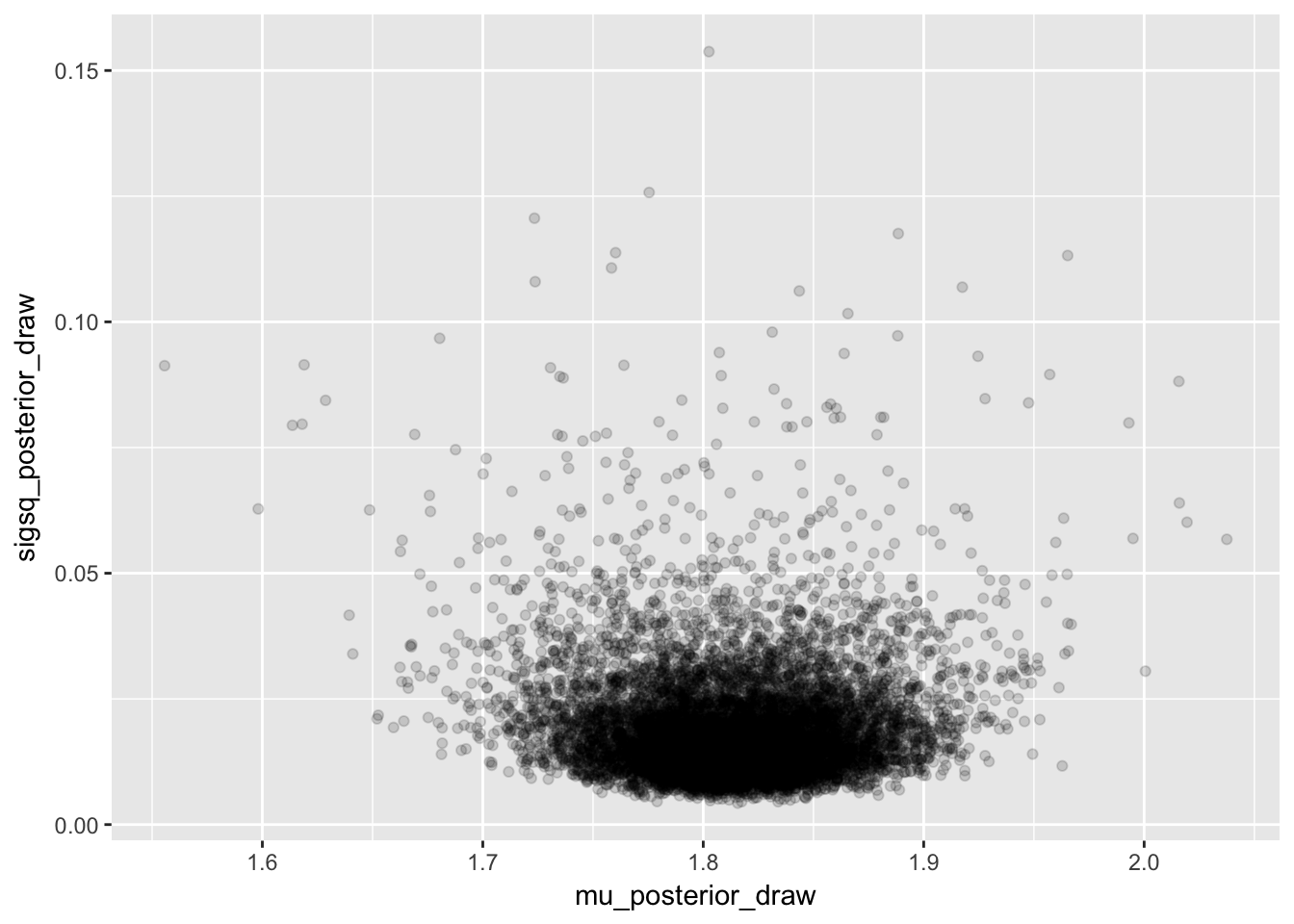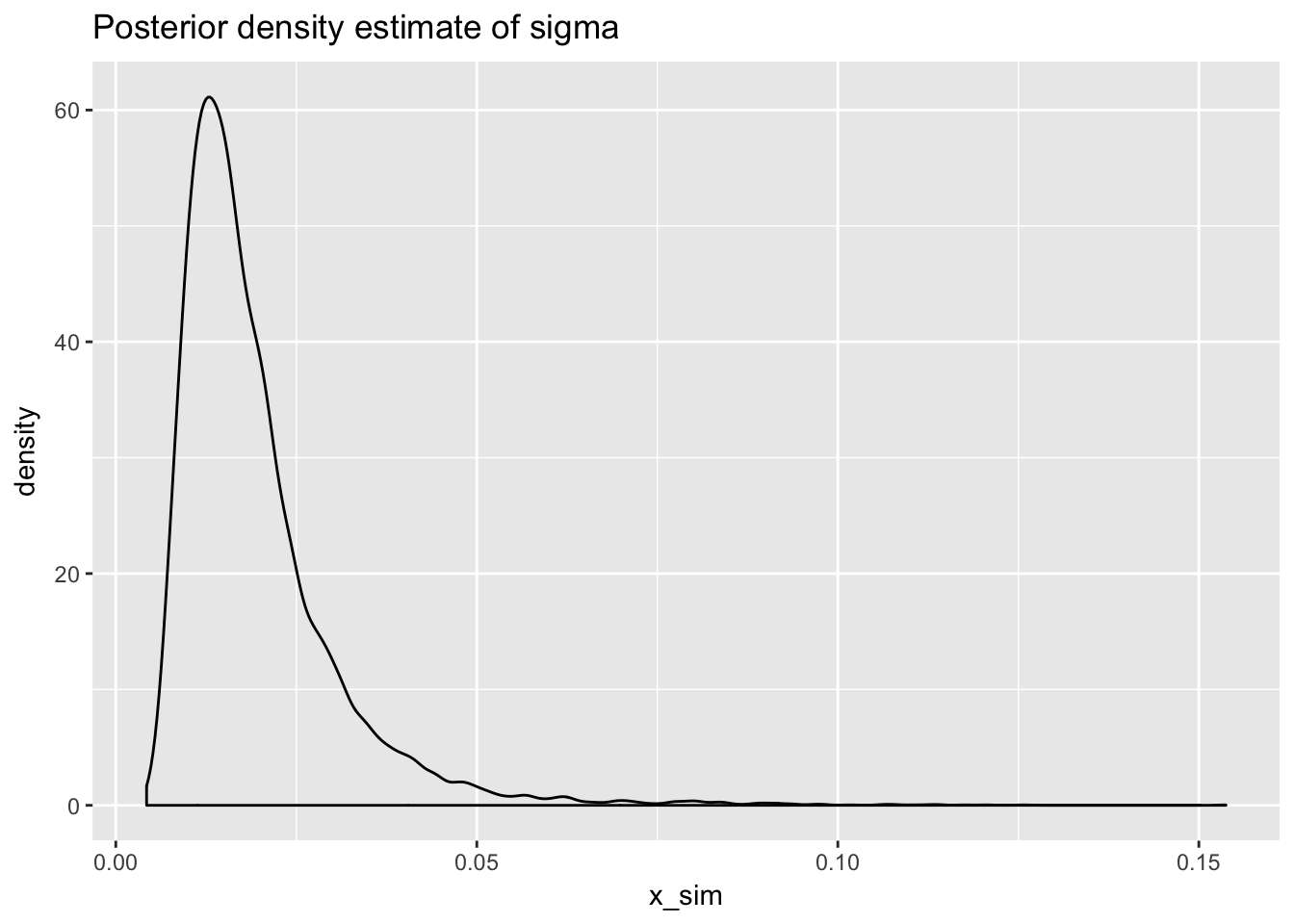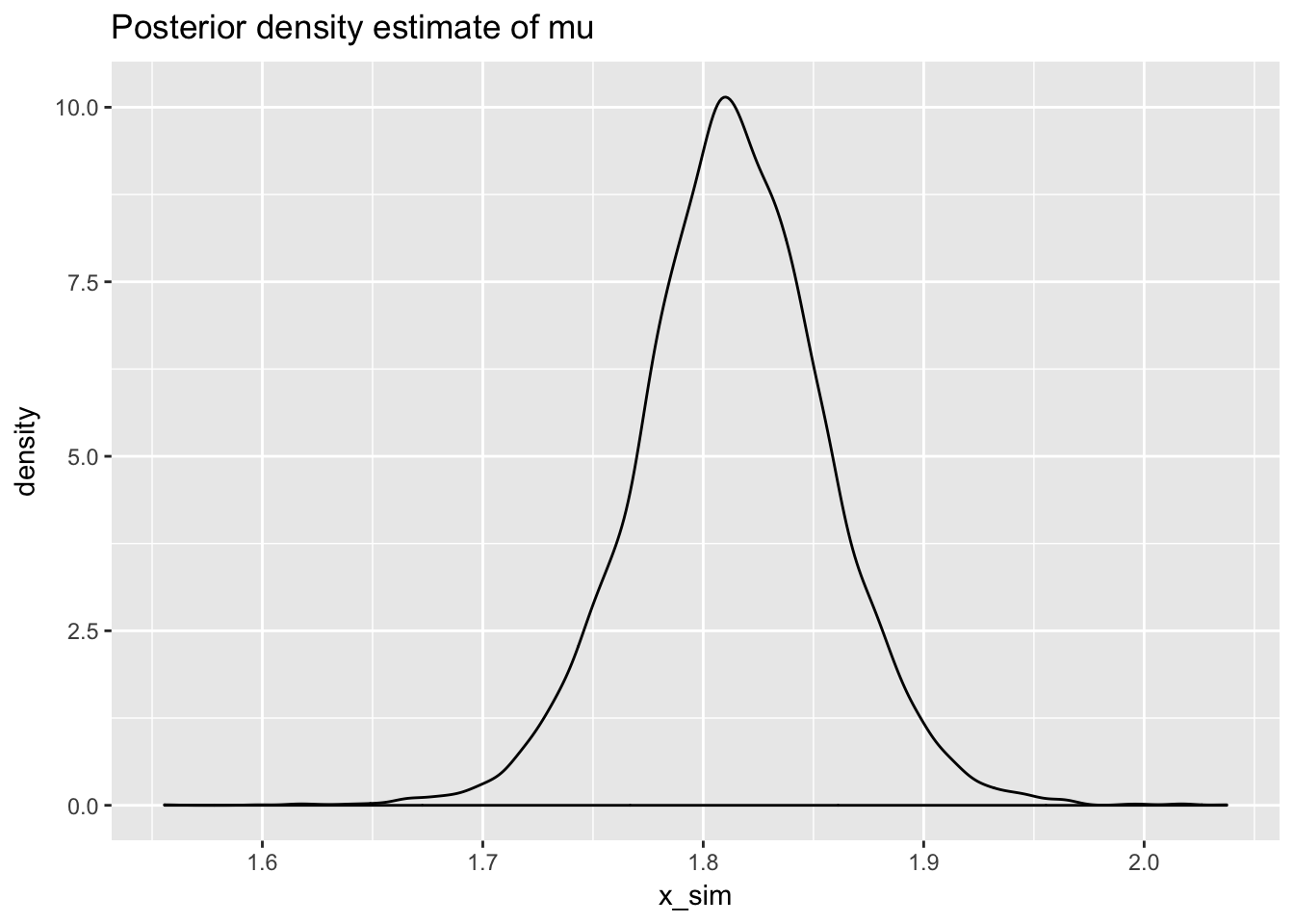# Bayesian Normal Model

### Inference for unknown mean and known variance

For a sampling model $$Y|\mu, \sigma^2 \stackrel{iid}{\sim} N(\mu,\sigma^2)$$ we wish to make inference on $$\mu$$ assuming $$\sigma^2$$ is known. The first step will be to derive an expression for $$\pi(\mu|Y=y,\sigma^2)$$ which is proportional to $$\pi(\mu|\sigma^2)\pi(y|\mu,\sigma^2)$$. To following prior structure will be adopted:

$Y|\mu,\sigma^2 \sim N(\mu,\sigma^2)$

$\mu \sim N(\mu_0,\sigma_0^2)$

Now we can derive an expression for $$\pi(\mu|Y=y,\sigma^2) \propto \pi(\mu|\sigma^2)\pi(y|\mu,\sigma^2)$$.

$\pi(\mu|\sigma^2)\pi(y|\mu,\sigma^2) \propto e^{-\frac{1}{2\sigma_0^2}(\mu-\mu_o)^2} e^{-\frac{1}{2\sigma^2} (y-\mu)^2}$

$= e^{\frac{1}{2}(\frac{1}{\sigma_0^2} (\mu^2-2\mu \mu_0 + \mu_0^2)+\frac{1}{\sigma^2}(y^2-2\mu y+\mu^2))}$

Now let’s just work with the $$\frac{1}{\sigma_0^2} (\mu^2-2\mu \mu_0 + \mu_0^2)+\frac{1}{\sigma^2}(y^2-2\mu y+\mu^2)$$ by itself.

$\frac{1}{\sigma_0^2} (\mu^2-2\mu \mu_0 + \mu_0^2)+\frac{1}{\sigma^2}(y^2-2\mu y+\mu^2)= \frac{\mu^2}{\sigma^2_0}-\frac{2\mu \mu_0}{\sigma^2_0} + \frac{\mu_0^2}{\sigma_0^2} + \frac{y^2}{\sigma^2} - \frac{2\mu y}{\sigma^2} + \frac{\mu^2}{\sigma^2}$

$= \mu^2(\frac{1}{\sigma^2_0} + \frac{1}{\sigma^2}) - 2\mu(\frac{\mu_0}{\sigma_0^2} + \frac{y}{\sigma^2}) + \frac{\mu_0^2}{\sigma_0^2} + \frac{y^2}{\sigma^2}$

To get this final expression into a quadratic form we can let $$a = \frac{1}{\sigma^2_0} + \frac{1}{\sigma^2}$$, $$b = \frac{\mu_0}{\sigma_0^2} + \frac{y}{\sigma^2}$$, and $$c = \frac{\mu_0^2}{\sigma_0^2} + \frac{y^2}{\sigma^2}$$. In proportionality the $$c$$ term will drop from the exponent. Now we have

$\pi(\mu | \sigma^2,y) \propto e^{-\frac{1}{2}(a\mu^2-2b\mu)} = e^{-\frac{1}{2}a(\mu^2 - 2b\mu/a+b^2/a^2)+b^2/2a}$

$= e^{-\frac{1}{2}a(\mu - b/a)^2} = e^{-\frac{1}{2}(\frac{\mu-b/a}{1/\sqrt{a}})^2}$

Which is recognizable as the kernel of a $$N(b/a,1/a) = N(\mu_n = \frac{\frac{\mu_0}{\sigma_0^2} + \frac{y}{\sigma^2}}{\frac{1}{\sigma^2_0} + \frac{1}{\sigma^2}}, \sigma^2_n = \frac{1}{\frac{1}{\sigma^2_0} + \frac{1}{\sigma^2}})$$.

### Inference for unknown mean and unknown variance

It is more reasonable to expect that in an actual data analysis if we are trying to estimate the mean of a normal population then we likely need to estimate the variance as well. The following parameterization is used by Hoff in A First Course in Bayesian Statistical Methods.

$1/\sigma^2 \sim gamma(shape = \frac{\nu_0}{2},rate = \frac{\nu_0 \sigma^2_0}{2})$

$\mu|\sigma^2 \sim N(\mu_0,\frac{\sigma^2}{\kappa_0})$

$Y_1,...,Y_n|\mu,\sigma^2 \stackrel{iid}{\sim} N(\mu,\sigma^2)$

The posterior joint distribution of $$\mu,\sigma^2$$ after observing the data $$Y_1,...,Y_n$$ is given by the following product.

$\pi(\mu,\sigma^2|y_1,...,y_n) = \pi(\mu|\sigma^2,y_1,...,y_n)\pi(\sigma^2|y_1,...,y_n)$

This first term $$\pi(\mu|\sigma^2,y_1,...,y_n)$$ is the conditional posterior distribution of $$\mu$$. Since it is conditional on $$\sigma^2$$ (i.e. $$\sigma^2$$ is “known”) it can be derived with a calculation identical to the one given in the previous section. Generalizing to a sample of size $$n$$ instead of size 1 and substituting $$\sigma^2/\kappa_n$$ for $$\sigma^2_0$$ we get that

$\mu|y_1,...,y_n,\sigma^2 \sim N(\mu_n = \frac{\kappa_0 \mu_0 + n\bar{y}}{\kappa_n},\frac{\sigma^2}{\kappa_n})$

where $$\kappa_n = \kappa_0 + n$$. Hoff’s parameterization lends itself to a nice interpretation of $$\mu_0$$ and the mean of $$\kappa_0$$ prior observations and $$\mu_n$$ as the mean of $$\kappa_0$$ prior observations and $$n$$ new observations all together. Also, the variance of $$\mu|y_1,...,y_n,\sigma^2$$ is $$\sigma^2/\kappa_n$$.

Now to get the posterior distribution of $$\sigma^2$$ after observing $$y_1,...,y_n$$ we need to integrate over all possible values of $$\mu$$ according to

$\pi(\sigma^2|y_1,...,y_n) \propto \pi(\sigma^2) \pi(y_1,...,y_n|\sigma^2)$

$= \pi(\sigma^2) \int_\mu \pi(y_1,...,y_n|\mu,\sigma^2)\pi(\mu|\sigma^2) d\mu$

$= \pi(\sigma^2) \int_\mu (\frac{1}{2\pi \sigma^2})^{-n/2} e^{-\frac{\sum(y_i-\mu)^2}{2\sigma^2}} (\frac{1}{2\pi \sigma^2/\kappa_0})^{-1/2} e^{-\frac{(\mu-\mu_0)^2}{2\sigma^2/\kappa_0}}d\mu$

Eventually, we get that $$\sigma^2|y_1,...,y_n \sim IG(\nu_n/2,\nu_n \sigma^2_n/2)$$ or alternatively $$1/\sigma^2|y_1,...,y_n \sim Gamma(\nu_n/2,\nu_n\sigma^2_n/2)$$, where

$\nu_n = \nu_0 + n$

$\sigma^2_n = \frac{1}{\nu_n}[\nu_0 \sigma^2_0 + (n-1)s^2+\frac{\kappa_0n}{\kappa_n}(\bar{y}-\mu_0)^2]$

We get another nice interpretation here of $$\nu_0$$ as the prior sample size with corresponding sample variance $$\sigma^2_0$$. Also $$s^2$$ is the sample variance and $$(n-1)s^2$$ is the sample sum of squares.

### normR

# devtools::install_github("carter-allen/normR")
library(normR)

### Monte Carlo Sampling

Example from Hoff pg. 76.

# parameters for the normal prior
mu_0 <- 1.9
kappa_0 <- 1

# parameters for the gamma prior
sigsq_0 <- 0.010
nu_0 <- 1

# some data
ys <- c(1.64,1.70,1.72,1.74,1.82,1.82,1.82,1.90,2.08)
n <- length(ys)
y_bar <- mean(ys)
ssq <- var(ys)

# posterior parameters
kappa_n <- kappa_0 + n
nu_n <- nu_0 + n
mu_n <- mu_n(kappa_0,mu_0,n,y_bar)
sigsq_n <- sigsq_n(nu_0,sigsq_0,n,ssq,kappa_0,y_bar,mu_0)
mu_n
##  1.814
sigsq_n
##  0.015324
# posterior variance draws
sigsq_posterior_draw <- draw_sigsq_posterior(10000,nu_n,sigsq_n)
# posterior mean draws
mu_posterior_draw <- draw_mu_posterior(mu_n,sigsq_posterior_draw,kappa_n)
quantile(mu_posterior_draw,c(0.025,0.975))
##     2.5%    97.5%
## 1.727323 1.899154
# plot posterior draws
normR::plot_monte_sim(sigsq_posterior_draw,mu_posterior_draw)# plot posterior density estimate for sigma
normR::plot_posterior_density_estimate(sigsq_posterior_draw,
plot_title = "Posterior density estimate of sigma")# posterior density estimate of mu
normR::plot_posterior_density_estimate(mu_posterior_draw,
plot_title = "Posterior density estimate of mu")### Shiny App

https://carter-allen.shinyapps.io/NormalModel/